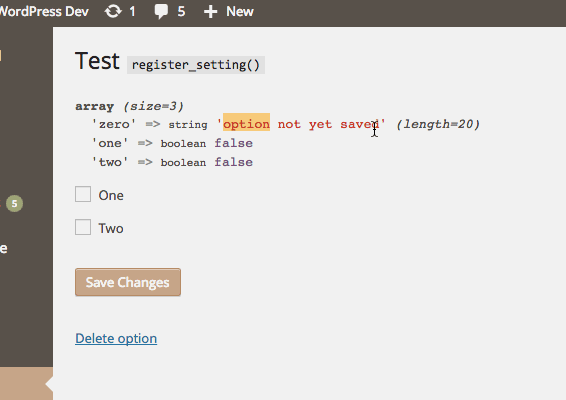# isset() Assumptions

`register_setting()` can be really handy, but take note! The first time your option gets saved, it’ll pass through the santize callback twice. With most input, it won’t matter; and if you’re explicit, it won’t matter either. But under the right conditions, it will.

Suppose your register_setting() looks like this:

``register_setting( \$option_group, 'checkbox', 'sanitize' );``

And you have a pair of checkboxes like this:

``````<input type="checkbox" name="checkbox[one]" value="1" />
<input type="checkbox" name="checkbox[two]" value="1" />``````

And finally, your sanitize callback looks like this

``````function sanitize( \$input ) {
\$input['one'] = isset( \$input[ 'one' ] ) ? true : false;
\$input['two'] = isset( \$input[ 'two' ] ) ? true : false;
return \$input;
}``````

See the problem?

On the very first save, both `\$input['one']` and `\$input['two']` will be true, no matter what. Say checkbox one is unchecked, when it fist passes through, `\$input['one']` is set to false – as expected. But when the input is passed through sanitize the second time, `\$input['one']` is set (to false) and thus the `\$input['one']` is changed to true.So the moral of the story is: be more explicit, don’t make assumptions.

## One thought on “isset() Assumptions”

1.John says:

This is why I prefer to use empty() instead of isset()